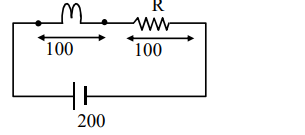# Solve this followingQuestion:

An electric bulb of 500 watt at 100 volt is used in a circuit having a $200 \mathrm{~V}$ supply. Calculate the resistance $R$ to be connected in series with the bulb so that the power delivered by the bulb is $500 \mathrm{~W}$.

1. $20 \Omega$

2. $30 \Omega$

3. $5 \Omega$

4. $10 \Omega$

Correct Option: 1

Solution:

500 watt at $100 \mathrm{v}$$\mathrm{P}=\mathrm{V}_{1}$

$500=V_{i}$

$\mathrm{i}=5 \mathrm{Amp}$

$\mathrm{V}=\mathrm{i} \times \mathrm{R}$

$\mathrm{R}=20$

Ans. 1